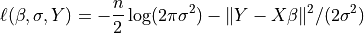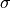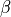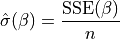Note

This page is a reference documentation. It only explains the class signature, and not how to use it. Please refer to the user guide for the big picture.

# nilearn.glm.OLSModel#

class nilearn.glm.OLSModel(design)[source]#

A simple ordinary least squares model.

Parameters:
designarray-like

This is your design matrix. Data are assumed to be column ordered with observations in rows.

Attributes:
designndarray

This is the design, or X, matrix.

whitened_designndarray

This is the whitened design matrix. design == whitened_design by default for the OLSModel, though models that inherit from the OLSModel will whiten the design.

calc_betandarray

This is the Moore-Penrose pseudoinverse of the whitened design matrix.

normalized_cov_betandarray

np.dot(calc_beta, calc_beta.T)

df_residualsscalar

Degrees of freedom of the residuals. Number of observations less the rank of the design.

df_modelscalar

Degrees of freedome of the model. The rank of the design.

Methods

 model.__init___(design) model.logL(b=self.beta, Y)
__init__(design)[source]#

Construct instance.

Parameters:
designarray-like

This is your design matrix. Data are assumed to be column ordered with observations in rows.

initialize(design)[source]#

Construct instance.

logL(beta, Y, nuisance=None)[source]#

Return the value of the loglikelihood function at beta.

Given the whitened design matrix, the loglikelihood is evaluated at the parameter vector, beta, for the dependent variable, Y and the nuisance parameter, sigma .

Parameters:
betandarray

The parameter estimates. Must be of length df_model.

Yndarray

The dependent variable

nuisancedict, optional

A dict with key ‘sigma’, which is an optional estimate of sigma. If None, defaults to its maximum likelihood estimate (with beta fixed) as sum((Y - X*beta)**2) / n, where n=Y.shape, X=self.design.

Returns:
loglffloat

The value of the loglikelihood function.

Notes

The log-Likelihood Function is defined asThe parameterabove is what is sometimes referred to as a nuisance parameter. That is, the likelihood is considered as a function of, but to evaluate it, a value ofis needed.

Ifis not provided, then its maximum likelihood estimate:is plugged in. This likelihood is now a function of onlyand is technically referred to as a profile-likelihood.

References

whiten(X)[source]#

Whiten design matrix.

Parameters:
Xarray

design matrix

Returns:
whitened_Xarray

This matrix is the matrix whose pseudoinverse is ultimately used in estimating the coefficients. For OLSModel, it is does nothing. For WLSmodel, ARmodel, it pre-applies a square root of the covariance matrix to X.

fit(Y)[source]#

Fit model to data Y.

Full fit of the model including estimate of covariance matrix, (whitened) residuals and scale.

Parameters:
Yarray-like

The dependent variable for the Least Squares problem.

Returns:
fitRegressionResults